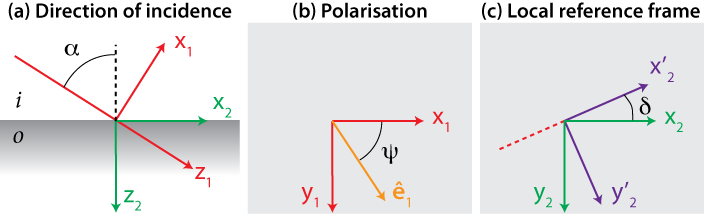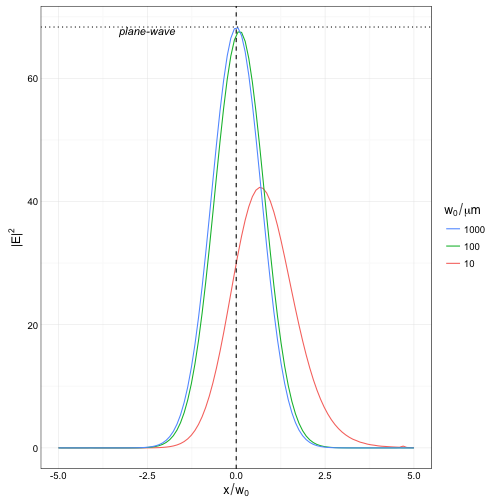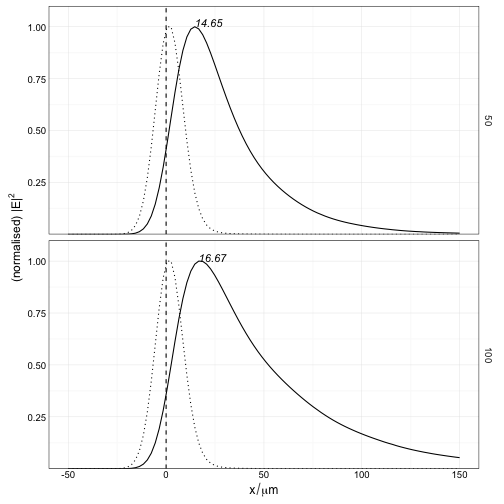# Near-field profile under gaussian-beam illumination

We consider a planar interface, possibly with multiple layers, illuminated by a gaussian beam from an incident medium ($$i$$). We seek to calculate the near-field profile in the outer medium ($$o$$).

### Description of the incident beamFollowing Novotny, we start with the angular spectrum representation of the incident beam with wavevector $$\mathbf{k}_i$$. In a frame $$F_1$$ attached to the central ray as depicted in Fig.~1, the electric field at a point $$\mathbf{r}_1$$, is expanded as (Novotny Eq. 3.9 p. 47, Eq. 3.27, p.54), $\mathbf{E}_1(\mathbf{r}_1) = \iint a(k_{i1x},k_{i1y}) \exp\left(i \mathbf{k}_{i1} \cdot \mathbf{r}_1 \right)\mathbf{\hat e}_1(\mathbf{r}_1,\mathbf{k}_{i1})\mathrm{d} k_{i1x}\mathrm{d} k_{i1y},$ where $a(\mathbf{k}_1) = \frac{w_0^2}{4\pi} e^{ -\frac{w_0^2}{4}(k_{1x}^2 + k_{1y}^2)}$ describes the gaussian field profile with waist $$w_0$$, and $$\mathbf{\hat e}_1 = \left(\cos \psi ; \sin \psi ; 0\right)^t$$ describes the electric field direction. Note that for a focused beam, the electric field direction would not be constant (see e.g Eq.~3 of Burghardt et al.), and the angular spectrum decomposition would contain a factor $$k/k_z$$ (close to 1 in the paraxial approximation).

### Rotation of reference frames

The transmitted electric field should be expressed in a reference frame attached to the planar interface (independent of the incident angle), we thus define a rotation matrix around the axis $$y_1$$. $R_y(\alpha) = \begin{bmatrix} \cos (\alpha) & 0 & \sin (\alpha)\ 0 & 1 & 0 \ -\sin (\alpha) & 0 & \cos (\alpha) \end{bmatrix}.$ For each individual plane wave in the integrand, a second rotation is performed around the $$z_2$$ axis, that brings the new reference frame $$(x'_2,y'_2,z'_2)$$ to coincide with the plane of incidence of that particular plane wave,

$R_z(\delta) = \begin{bmatrix} \cos (\delta) & \sin (\delta) & 0\ -\sin (\delta) & \cos (\delta) & 0 \ 0 & 0 & 1 \end{bmatrix}.$

The angle of rotation $$\delta$$ is given by

$\delta = \sin^{-1}\frac{s_{2y}}{\sqrt{s^2_{2x} + s^2_{2y}}}$ where $$\mathbf{\hat s}_2 = R_y(\alpha) \mathbf{\hat s}_1$$ is obtained by rotation of the normalised incident wavevector $$\mathbf{\hat s}_1 = \mathbf{k}_{i1}/|\mathbf{k}_{i1}|$$. Each plane wave, expressed in this dedicated frame of reference, is now written

$\mathbf{E}_{i2'}(\mathbf{r}_{2'}) = \mathbf{\hat e}_{i2'}\exp\left(i \mathbf{k}_{i2'} \cdot \mathbf{r}_{2'} \right).$

### Transmission at the interface

We consider an individual plane wave incident on the interface, and express the amplitude in the frame $$F_2'$$ of the electric field $$\mathbf{E}_{o2'}$$ on the outer side using the Fresnel coefficients $$t^p$$ and $$t^s$$ (Etchegoin, Le Ru, App. F.3),

$\mathbf{E}_{o2'} (\mathbf{r}_{2'}) = \begin{bmatrix} \left(\frac{n_i}{n_o}\right)^2\frac{k_{o2z}}{k_{i2'z}}t^p E_{i2'x}\ t^s E_{2'y}\ \left(\frac{n_i}{n_o}\right)^2t^p E_{i2'z} \end{bmatrix}\exp\left(i \mathbf{k}_{o2'}\cdot \mathbf{r}_{2'} \right).$ The wave vector of the transmitted, potentially evanescent plane wave is given by $$\mathbf{k}_{o2'} = \left(k_{i2'x}, k_{i2'y}, \sqrt{k_o^2 - (k_{i2'x}^2+k_{i2'y}^2)}\right)$$.

The electric field is finally transformed back into the reference frame $$F_{2}$$ by a rotation of $$R_z(-\delta)$$.

The integration over the distribution of incident plane waves is performed in polar coordinates,

$\mathbf{E}_{o2}(\mathbf{r}_2) = \int_0^{2\pi} \int_0^1 a(\rho,\theta) \mathbf{E}_{o2} (\mathbf{r}_{2}, \rho, \theta) \rho k_i^2 \mathrm{d}\rho \mathrm{d} \theta,$

with

\left\{\begin{aligned} k_{ix1} &=k_i \rho\cos\theta\ k_{iy1} &=k_i \rho\sin\theta\ \end{aligned}\right.. In practice, and to reduce the computation time, the range of integration for $$\rho$$ is restricted to $$[0, 6/(k_i w_0)]$$; this cutoff value for $$\rho$$ was chosen such that the corresponding weight factor $$e^{ -\frac{w_0^2}{4}k_i^2\rho^2}$$ in the integrand is reduced by a factor $$\exp(-3^2)\sim 10^{-4}$$ compared to the central ray.

### Numerical implementation and code considerations

The code is split into 2 main functions,

colvec integrand_gb_layer(const colvec& rt,
const colvec& r2,
const double ki,
const double psi,
const double alpha,
const double w0,
const double ni,
const double no,
const cx_double nl,
const double d)


is the integrand, that calculates the transmitted electric field at a point r2(x,y,z) given a value of $$rt = (\rho, \theta)$$, and the parameters of the system. The complex electric field is reshaped into a 6-vector with real components (interlaced) suitable for 2D adaptive numerical quadrature (routine \texttt{hcubature}). The integration routine is called sequentially for $$N$$ points r2 in the function

cx_mat field_gb_layer(const mat& r2,
const double k0,
const double psi,
const double alpha,
const double w0,
const cx_vec& epsilon,
const vec& thickness,
const int maxEval,
const double reqAbsError,
const double tol,
bool progress)


returning a $$N\times 3$$ complex matrix of electric fields. If points in r2 lie before the interface (negative z), the electric field is calculated as the sum of the reflected and incident fields. It should be noted, however, that points lying inside the multilayer structure ($$0integrand_gb_ml and field_gb_ml, calling multilayer_field for the transfer matrix calculation, at the expense of a computional time (\(\sim 1.5$$ times slower).

### Validation of the results

Surprisingly few results are available in the literature. We can first check that the limit of large beam waist agrees with the simpler case of plane-wave illumination.A similar calculation was also performed by Weeber et al. (Phys Rev B 83, 115433 (2011)), though they used a simplified 1D parametrisation of the gaussian beam, thus neglecting saggital (“off-axis”) rays. Because SPPs are only excited with TM-polarised light, however, their influence on the results is minimal. Below we reproduce the beam-shift discussed in their work.